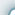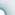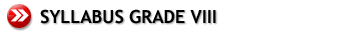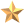Coming Soon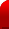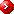New Elementary Mathematics 2 – (VIII Grade)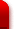IndicesAlgebraic ManipulationsLiteral And Quadratic Equations Positive Integral Indices Zero and Negative Integral Indices Indices Involving Variables Standard Form Special Algebraic Rules for Calculations Expanding Products Factorisation Addition and Subtraction of Algebraic Fractions Multiplication and Division of Algebraic Fractions Fractional Equations Problems Involving Fractional Equations Literal Equations Transformation of Formulae Quadratic Equations Quadratic Factorisation Solving Quadratic Equations by Factorisation Solving Quadratic Equations by Factorisation Word Problems Leading to Quadratic EquationsWord Problems Graphs I Simultaneous Linear Equations Problems on Rate, Ratio and Percentage Problems Involving Financial Transactions The Coordinate Plane Graphs of Linear Equations in Two Variables Simple Applications of Straight Line Graphs Travel Guide Solving Simultaneous Linear Equations by Graphs Quadratic Graphs Simple Elimination Method Elimination Method Substitution Method Word Problems Using Simultaneous EquationsInequalities Congruent and Similar Triangles Mensuration Sets of Numbers on the Number Line Properties of Order Solving Inequalities Congruent Triangles Similar Triangles Sectors Cones and Pyramids Sphere Areas of Similar Figures Volumes of Similar SolidsPythagoras' Theorem and Trigonometry Motion Geometry Statistics I Pythagoras' Theorem Indirect Measure Trigonometrical Ratios Trigonometrical Ratios by Using a Calculator Solution of Right-angled Triangles Applications of Trigonometrical Ratios to Simple Problems Reflection Rotation Translation Enlargement Combination of Transformations Collection and Presentation of Data Reading and Drawing of Bar Graphs Reading and Drawing of Pie Charts Reading and Drawing of Line Graphs and PictogramsStatistics II More Algebraic Manipulations Frequency Distribution Mean, Mode and Median of Frequency Distributions Types of Averages Addition and Subtraction of Polynomials Multiplication of Polynomials Division of Polynomials (Optional) Algebraic Fractions and Fractional Equations Algebraic Manipulation Manipulation of Expressions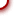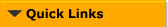cybeRShala Difference Lesson Illustration How It Works Compare Us Request Info Placement Test Tell a friend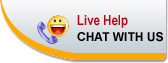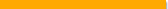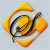Home | FAQ | Tell a Friend | Contact Us | Compare Us | Homeschool Resources | Sitemap cybershala.com Inc. All Rights reserved | Terms of service | Privacy policy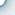s